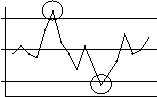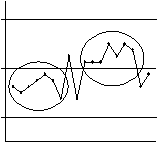The Psychology of Quality and More

# Control Chart: How to understand it

The Quality ToolbookControl Chart > How to understand it

## How to understand it

When a process is run repeatedly, even under apparently stable conditions, measurements made of it will seldom be identical. A Histogram can be used to show the static distribution of a set of these measurements, but this does not show dynamic trends, for example where successive measurements may indicate a significant change within the process (Fig. 1).Fig. 1. Concealed dynamics of Histogram

A Control Chart usually has three horizontal lines in addition to the main plot line, as shown below (Fig. 2). The central line is the average (or mean). The outer two lines are at three standard deviations either side of the mean. Thus 99.7% of all measurements will fall between these two lines.Fig. 2. Mean and Control Limits

The top line is called the upper control limit and the bottom line is called the lower control limit, as points falling outside these bounds are considered as being out of control. These limits are often abbreviated to UCL and LCL. Although control limits are straight lines for most types of Control Charts, there are some types of chart where they are different for each plotted point, as illustrated.

Note that specification limits are not related to control limits. Specification limits say what the process results should be, whilst control limits show what the process is actually doing.

The actual calculations of control limits varies with the type of measurement being portrayed (this is for mathematical reasons). Measurements which answer the question, 'How much?' are called variables (e.g. weight, time, voltage). A further identification is that they are measured in quantitative units, such as grams and seconds. Non-variable measurements are called attributes. These ask, 'How many?', measuring countable items, such as the number of defective items or the number of actual defects in a batch (note the difference: one defective item may contain several defects).

Each point on a variables Control Chart is usually made up of the average of a set of measurements. This is for two reasons.

Firstly, it results in a predictable Normal (bell-shaped) distribution for the overall chart, due to the Central Limit Theorem.

Secondly, this will result in tighter control limits. This is because the averaging effect in each group smooths out individual high and low measurements, resulting in a Control Chart that can detect smaller changes in the process than one which plots one point for each measurement. This is illustrated in Fig. 3, which shows how the same shift in average results in a greater likelihood that a narrow distribution will detect this change. In a Control Chart where each point represents the average of a set of measurements, this would result in points outside the control limits that would not be outside the limits of a Control Chart where each point represents a single measurement.Fig. 3. How control limits catch shifts

Two Control Charts must be drawn when tracking variables, because just measuring the average of subgroups could result in significant variation within the subgroups being missed, as illustrated below. It may also be noticed here that the control limits for the range chart are not symmetrical about the center average line. This is because although the average chart has a Normal distribution, the distribution of the range chart is skewed. It is also common for the lower control limit of a range chart to be on the zero line, as a negative value would be nonsense.Fig. 4. Variation within sub-groups

Interpretation of the Control Chart requires identification of significant factors such as points which fall outside the control limits or patterns which repeat seven or more times. A significant point or pattern will indicate that the process is 'out of control'. This does not necessarily mean that it is wildly wrong, but it does indicate that it is not statistically stable. Conversely, a statistically stable process may have unsatisfactorily wide variation.

When significant patterns or points are found, then assistance with identifying possible typical causes may be found by using a Cause-Effect Diagram. These include changes in people, the actions they carry out, the tools and machines used, the raw materials and the general environment. Basic patterns to look for and possible interpretations are shown in Table 1.

Table 1. Significance in Control Charts

 Control Chart Name Description Possible interpretationSpecial cause of variation Point outside control limits. Something unusual has happened. e.g. Person was interrupted.Shift Seven or more consecutive points, all on one side of the central average line. The overall average has changed. e.g. Showing result of process improvement.Trend Seven or more consecutive points, all increasing or decreasing in value. Gradual change in the process. e.g. A tool is wearing out.Cycle Seven or more repeating patterns (possibly over several points). Time-related effect. e.g. People changing with shifts.

### You can buy books here

And the big
paperback book